Calculation of reactions in beam supports

For a given two-support beam with a cantilever part loaded with a load complex: force F, moment m and distributed load q, determine the magnitude and direction of the supporting reactions.

The design scheme of the beam is shown in Fig. 1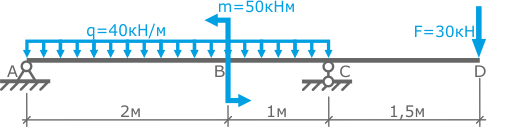Fig. 1

The span of the beam is 3 m. The length of the console part is 1.5 m.

Example solution

We recommend you to watch our video tutorial on the calculation of the supporting reactions of the beam:

Video by subject (contains English subtitles)

To solve the problem, we denote the characteristic points (sections) of the beam (points A, B, C and D) and determine the position of the coordinate system y-z, choosing its origin for example in volume A (Fig. 2)Fig. 2

Both beam supports are articulated, therefore in each of them only force will arise, we will designate them accordingly RA and RC

Since all the given loads are located exclusively in the vertical plane (plane transverse bending) and do not give projections to the z axis, then the support reactions will also be only vertical.

Generally speaking, the reactions in the supports are such forces that are necessary to keep the beam with the loads applied to it, in a static (stationary) state. In this case, these forces do not allow it to rotate and move in a vertical plane.

This beam is statically definable, because Equilibrium equations are sufficient to determine the unknown forces in the beam supports.

To compose the equations of statics, the reference reactions RA and RC are preliminarily sent arbitrarily, for example, upwards (Fig. 3).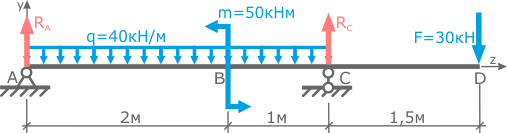Fig. 3

Two equations will be needed to determine the two unknown reactions.

We write down the equations of statics:

1. The beam does not move vertically, i.e. the sum of the projections of all forces on the y axis is zero: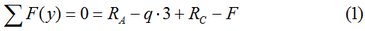Here, according to the rule of signs for the projection of forces on the axis, the loads of which coincide with the positive direction of the y axis are written positive and vice versa.
2. The fact that the beam does not rotate indicates that the sum of the moments relative to any of its points is also zero, that is: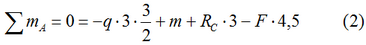In this equation, according to the rules of signs for moments, concentrated forces, moments and distributed loads, those seeking to turn the beam counterclockwise relative to the considered point A are written positive and vice versa.
How to record the moment of distributed load is shown here.
The force applied at a point relative to which the sum of the moments in the equation is considered is not involved, since the moment arm for it is zero.

Here, the sum of the moments is better recorded relative to the point located on the support (for example, A), because in this case, the corresponding reaction RA in the equation is not involved.

From the expression (2) we determine RC:and substituting it into the expression (1) we find RA: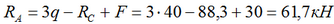The direction and magnitude of the reactions are usually necessary for further calculations of the beam for strength and rigidity, therefore, in order to avoid possible errors, it is recommended to perform a check of the found values.